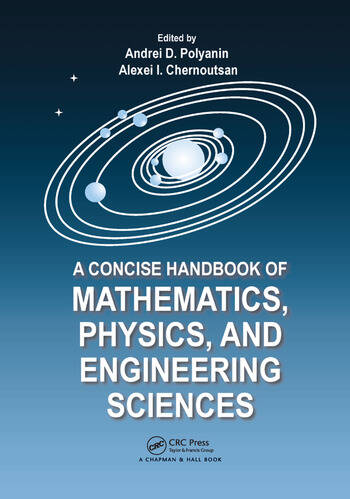# A Concise Handbook of Mathematics, Physics, and Engineering Sciences

## 1st Edition

Andrei D. Polyanin, Alexei I. Chernoutsan

CRC Press
Published November 13, 2017
Reference - 1125 Pages - 381 B/W Illustrations
ISBN 9781138199040 - CAT# K31436

For Instructors Request Inspection Copy

was \$61.95

USD\$49.56

SAVE ~\$12.39

FREE Standard Shipping!

## Preview

### Summary

A Concise Handbook of Mathematics, Physics, and Engineering Sciences takes a practical approach to the basic notions, formulas, equations, problems, theorems, methods, and laws that most frequently occur in scientific and engineering applications and university education. The authors pay special attention to issues that many engineers and students find difficult to understand.

The first part of the book contains chapters on arithmetic, elementary and analytic geometry, algebra, differential and integral calculus, functions of complex variables, integral transforms, ordinary and partial differential equations, special functions, and probability theory. The second part discusses molecular physics and thermodynamics, electricity and magnetism, oscillations and waves, optics, special relativity, quantum mechanics, atomic and nuclear physics, and elementary particles. The third part covers dimensional analysis and similarity, mechanics of point masses and rigid bodies, strength of materials, hydrodynamics, mass and heat transfer, electrical engineering, and methods for constructing empirical and engineering formulas.

The main text offers a concise, coherent survey of the most important definitions, formulas, equations, methods, theorems, and laws. Numerous examples throughout and references at the end of each chapter provide readers with a better understanding of the topics and methods. Additional issues of interest can be found in the remarks. For ease of reading, the supplement at the back of the book provides several long mathematical tables, including indefinite and definite integrals, direct and inverse integral transforms, and exact solutions of differential equations.

#### Instructors

We provide complimentary e-inspection copies of primary textbooks to instructors considering our books for course adoption.